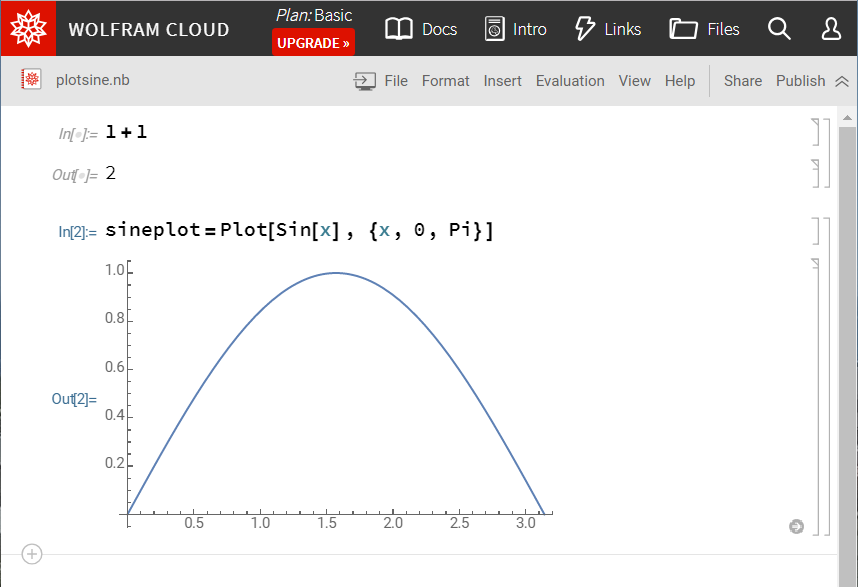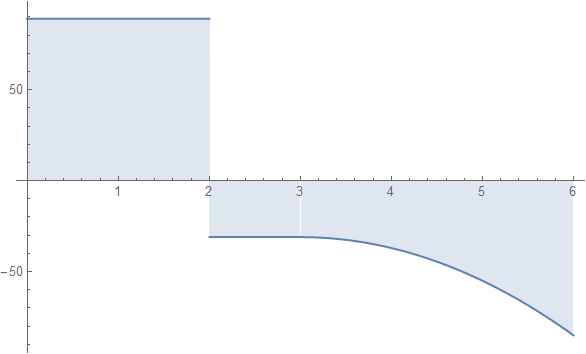# Computational Biomechanics

Dr. Kewei Li

## Mathematica and Wolfram Cloud

### Introduction

Mathematica is a very powerful scientific computing program which has been used in many scientific and engineering fields. It encompasses computer algebra, symbolic and numerical computation, visualization, and statistics capabilities. The Wolfram Research company has also developed a computer programming language called the Wolfram language besides Mathematica. The Wolfram language is a powerful language for interactive computing often times with Mathematica as the front end. You can try it immediately on the web in the Wolfram Language Sandbox or register for a basic account at the Wolfram cloud for free.You may have also used the Wolfram Alpha which claims to be a computational knowledge engine and can also be used to quickly plot some functions.

The back end of all those products is the Wolfram Engine which is now available free for developers. Since the Mathematica license is not “freely” available for all the students at TU Graz, you are highly recommended to try out the free Wolfram engine if you are not satisfied with the free account of Wolfram cloud. For example, you can use the Wolfram engine to plot some functions for your homework and export it as a PDF or picture file.

### Some Examples

Here is an example to plot the bending-moment diagram from a beam bending problem,

Plot[Piecewise[{ {89, 0 < x < 2}, {-31, 2 < x < 3},
{-85 + 36 x - 6 x^2, 3 < x < 6} }], {x, 0, 6},
Filling -> Axis]


and the output,Go Back